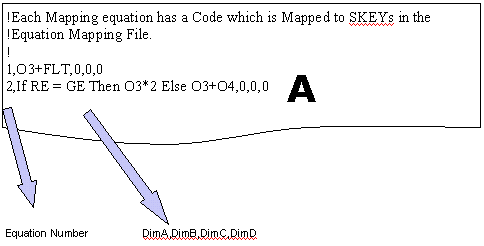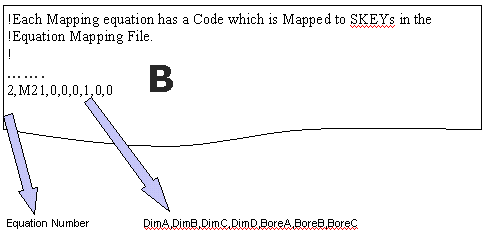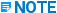# Map Equations file - Intergraph I-Convert - Help - Intergraph Process, Power & Marine Documentation

## SmartPlant Isometrics I-Convert Help

PPMProduct
Intergraph I-Convert
PPMCategory_custom
Help
Version_IConvert
7.1(2014R1)

The Map Equations file contains equations that match the settings in the Elbolet data file. The following examples illustrate the contents of the Map Equiations files for PDS (A) and PDMS (B).During a default installation of the software, sample PDMS-MapEquations.txt and PDS-MapEquations.txt files are delivered to the <installation drive>:\Program Files\SmartPlant\Isometrics\I-Convert\Samples folder.

PDS information

When using PDS, you are allowed to create physical data files with dimensions in whatever columns you want. However, because the dimensions required in the catalog sometimes need to be calculated from data in the PDS files rather than simply read from a given column in the file, it is necessary to specify the appropriate mapping between the columns of data in the PDS files and the SmartPlant Isometrics component dimensions. This mapping can be a pointer to a column in the file, a calculation performed on data from a specified column, a logical evaluation based on data in the PCD file to select the appropriate column, or a combination of any of these. For more information, see Review PDS component data and Layout and content of the PCD file in PDS Technical Information.

Each mapping equation has a code that is mapped to SKEYs in the Equation Mapping file. The value of the Dimension Mapping in the I-Convert project file, specifies which mapping to use. The format of each entry is shown in the following example :

EquationCode,Data for DimA,Data for DimB,Data for DimC,Data for DimD

• EquationCode is an integer starting at 1. Entries must be in EquationCode order.

• The four data fields can contain the following: O1 through O9, which indicates that the value held in output column 1 through 9 should be assigned to the dimension. A, B, C, or D, which indicates that this dimension should be set equal to the specified dimension DimA, DimB, DimC or DimD.

An equation, such as O2/2+O3, which indicates that the dimension is given by output 3 plus a half of output 2.

There is also a special operator, Tan, which is interpreted as the Tangent of (Bend Angle in Radians)/2.

In the case of flanges, the overall length sometimes consists of two parts. The first is the thickness of the flange. The second is the length of the remainder, such as the hub length for a weld neck flange. The dimension can be calculated as the length in O3 plus the flange thickness, which is specified by the special variable FLT, as shown in the following example:

FLWN,O3+FLT,0,0,0If you need to use the pipe OD in an equation, the special variable HOD can be used, which equates to half the outside diameter (BoreA). The following shows the syntax for that equation: 0,0,HOD+O2,0.

A conditional statement which indicates that the dimension is found in different places depending on whether, for example, the inlet and outlet end types are equal (RE = GE) or the inlet and outlet bores are equal (BA = BB), or a specified output column has a zero value (O4 = 0). A zero which indicates that this dimension is not mapped to an output column.

The OLET code is used to identify the whole range of OLET type branch fittings.

The DEF code identifies the default mapping for any items not specifically mapped.

PDMS information

PDMS uses point sets to specify how the various dimensions of the components are held. For more information, see PDMS technical information.

The data extracted from PDMS by CatScan holds its dimensional information as a series of point locations relative to a notional origin of the component. Each point can also have a nominal size. For more information, see PDMS technical information.

I-Convert contains internal default mapping based on the SmartPlant Isometrics component configuration, but point sets can be user-defined, making it is possible to override the internal mapping and specify the relationship between data extracted for the various points and the dimensions and bores required in the catalog in user defined mapping equations.

Each mapping equation has a code that is mapped to SKEYs in the SKEY table. The Dimension Mapping value in the I-Convert project file specifies this and must be set to:

Dimension Mapping = SKEY

The format of each entry is EquationCode,Data for DimA,Data for DimB,Data for DimC,Data for DimD,Data for BoreA,Data for BoreB,Data for BoreC, as shown in the following example:

1,P1,P2,M21,0,1,2,0

The EquationCode is an integer starting at 1. Entries must be in EquationCode order. The first four data fields contain the mapping for the four dimensions in order. Each must contain one of the following:

• P1 through P6, where P1 (P2, P3, P4, and so forth) indicates that the absolute value of PDis1 (PDis2, PDis3, and so forth) should  be assigned to this dimension, and so forth. PDis1 is the absolute value of the distance from the PDMS component origin (Pt0) to the specified point (Pt1).

• M21, which indicates that the dimension should be set to P2*SignP2 - P1*SignP1. If Pt2 is 5 units in the positive direction with respect to Pt0, and Pt1 is 5 units in the negative direction with respect to Pt0, the dimension is computed as (5 (+1))-(5*(-1)) = 10.

• S3, which indicates that the dimension should be set to P3*SignP3. This form is used for TEED fittings where the value of the leg length used by SmartPlant Isometrics is often negative. As an example, if a TEED elbow has it branch connect point -5 units in the positive direction from Pt0, the dimension is computed as -5*(+1) = -5.Similarly, if its position was given as 5 units in the negative direction with respect to Pt0, the dimension would be computed as 5*(-1) = -5.

• X21, Y21, Z21, Z31, and so forth, where X21 (and so forth) indicates that the dimension should be set to the incremental movement between Pt2 and Pt1 (and so forth) in the X direction.

• A 0 (zero), which indicates that this dimension is not used.

The last three data fields contain the mapping for the three bores in order. Each must contain a single digit 0 through 7. 0 signifies that this bore is not set, 1 signifies that this bore should be set to the Bore of Pt1, 2 signifies that this bore should be set to the Bore of Pt2, and so forth.